# Magnetic monopole

In physics, the phrase "magnetic monopole" usually denotes a Yang–Mills potentialand Higgs fieldwhose equations of motion are determined by the Yang–Mills–Higgs actionIn mathematics, the phrase customarily refers to a static solution to these equations in the Bogomolny–Prasad–Sommerfield limit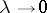which realizes, within its topological class, the absolute minimum of the functional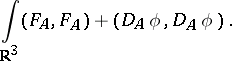This means that it is a connectionon a principal-bundle over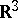(cf. also Connections on a manifold; Principal-object) and a sectionof the associated adjoint bundle of Lie algebras such that the curvatureand covariant derivativesatisfy the Bogomolny equations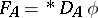and the boundary conditions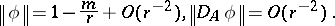Pure mathematical advances in the theory of monopoles from the 1980s onwards have often proceeded on the basis of physically motivated questions.

The equations themselves are invariant under gauge transformations and orientation-preserving isometries. Whenis large,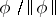defines a mapping from a-sphere of radiusinto an adjoint orbit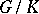and the homotopy class of this mapping is called the magnetic charge. Most work has been done in the case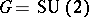, where the charge is a positive integer. The absolute minimum value of the functional is then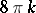and the coefficientin the asymptotic expansion of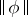is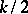.

The first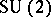solution was found by E.B. Bogomolny, M.K. Prasad and C.M. Sommerfield in 1975. It is spherically symmetric of chargeand has the form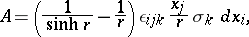In 1980, C.H. Taubes [a10] showed by a gluing construction that there exist solutions for all largerand soon after explicit axially-symmetric solutions were found. The first exact solution in the general case was given in 1981 by R.S. Ward for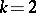in terms of elliptic functions.

There are two ways of solving the Bogomolny equations. The first is by twistor methods. In the formulation of N.J. Hitchin [a6], an arbitrary solution corresponds to a holomorphic vector bundle over the complex surface, the tangent bundle of the projective line. This is naturally isomorphic to the space of oriented straight lines in. The boundary conditions show that the holomorphic bundle is an extension of line bundles determined by a compact algebraic curve of genus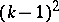(the spectral curve) in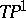, satisfying certain constraints. The second method, due to W. Nahm [a12], involves solving an eigenvalue problem for the coupled Dirac operator and transforming the equations with their boundary conditions into a system of ordinary differential equations, the Nahm equations,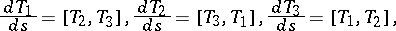where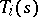is a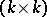-matrix-valued function on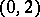. Both constructions are based on analogous procedures for instantons, the key observation due to N.S. Manton being that the Bogomolny equations are dimensional reductions of the self-dual Yang–Mills equations (cf. also Yang–Mills field) in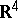. The equivalence of the two methods forand their general applicability was established in [a7] (see also [a8]). Explicit formulas forandare difficult to obtain by either method, despite some exact solutions of Nahm's equations in symmetric situations [a5].

The case of a more general Lie group, where the stabilizer ofat infinity is a maximal torus, was treated by M.K. Murray [a11] from the twistor point of view, where the single spectral curve of an-monopole is replaced by a collection of curves indexed by the vertices of the Dynkin diagram of. The corresponding Nahm construction was described by J. Hurtubise and Murray [a9].

The moduli space (cf. also Moduli theory) of allmonopoles of chargeup to gauge equivalence was shown by Taubes [a14] to be a smooth non-compact manifold of dimension. Restricting to gauge transformations that preserve the connection at infinity gives a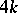-dimensional manifold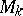, which is a circle bundle over the true moduli space and carries a natural complete hyper-Kähler metric [a1] (cf. also Kähler–Einstein manifold). With respect to any of the complex structures of the hyper-Kähler family, this manifold is holomorphically equivalent to the space of based rational mappings of degreefrom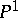to itself [a3]. The metric is known in twistor terms [a1], and its Kähler potential can be written using the Riemann theta-function of the spectral curve [a8], but only the caseis known in a more conventional and usable form [a1] (as of 2000). This Atiyah–Hitchin manifold, the Euclidean Taub–NUT metric andare the only-dimensional complete hyper-Kähler manifolds with a non-triholomorphicaction. Its geodesics have been studied and a programme of Manton concerning monopole dynamics put into effect. Further dynamical features have been elucidated by P.M. Sutcliffe and C.J. Houghton [a15] using a mixture of numerical and analytical techniques.

A cyclic-fold covering ofsplits isometrically as a product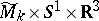, where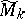is the space of strongly centred monopoles. This space features in an application of-duality in theoretical physics, and in [a13] G.B. Segal and A. Selby studied its topology and theharmonic forms defined on it, partially confirming the physical predictions.

Magnetic monopoles on hyperbolic three-space were investigated from the twistor point of view by M.F. Atiyah [a2] (replacing the complex surfaceby the complement of the anti-diagonal in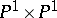) and in terms of discrete Nahm equations by Murray and M.A. Singer, [a16].

How to Cite This Entry:
Magnetic monopole. Encyclopedia of Mathematics. URL: http://encyclopediaofmath.org/index.php?title=Magnetic_monopole&oldid=24160
This article was adapted from an original article by N.J. Hitchin (originator), which appeared in Encyclopedia of Mathematics - ISBN 1402006098. See original article